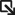• Solutions Solutions
• Products Products
• Services Services
• Support SupportSupport Portal ›
• Resources Resources
##### Networking Made Simple, Easy, and Affordable - Q&A with the Senior Director of Extreme Capital Solutions
All stories ›
• Partners Partners

# Delta Expression

A CLEAR-Flow delta expression computes the difference from one sample to the next of a counter value.

This difference is compared with the threshold value. The following is the syntax for a CLEAR-Flow delta expression:

```delta <counterName> REL_OPER <countThreshold> ;
hysteresis <hysteresis> ;
```

The values of countThreshold and hysteresis can be specified as floating point numbers. The delta expression specifies how to compare the difference in a counter value from one sample to the next with its threshold. The counterName is the name of an ACL (Access Control List) counter referred to by an ACL rule entry and the countThreshold is the value compared with the difference in the counter from one sample to the next. The REL_OPER is selected from the relational operators for greater than, greater than or equal to, less than, or less than or equal to (>, >=, <, <=).

The hysteresis hysteresis statement is optional and sets a hysteresis value for the threshold. After the delta statement is true, the value of the threshold is adjusted so that a change smaller than the hysteresis value will not cause the statement to become false. For statements using the REL_OPER > or >=, the hysteresis value is subtracted from the threshold; for < or <=, the hysteresis value is added to the threshold.

For example, the following delta expression:

```delta counter1 >= 100 ;
hysteresis 10 ;
```

will only be true after the delta of the counter reaches at least 100. At the time it becomes true, the hysteresis value is subtracted from the threshold (setting the threshold to 90). With the threshold now at 90, the condition would stay true until the delta of the counter becomes less than 90.

If the expression becomes false, the threshold is reset to its original value. You would use the hysteresis value to prevent the expression from vacillating between the true and false states if the difference between the counter values is near the threshold. If the hysteresis value is greater than the threshold value, the hysteresis value will be set to 0.

The following table is an example of evaluating the CLEAR-Flow delta expression above multiple times. Notice that the rule is not triggered until evaluation 4, when the delta value (the change in the counter value from one evaluation to the next) is greater than or equal to 100. After the rule is triggered, it remains triggered until the delta value is less than 90 (the original threshold minus the hysteresis), at evaluation 7. At evaluation 9, the rule is again triggered when the delta reaches 100. The rule will remain triggered until the delta drops below 90.Delta Expression Evaluation Example

Evaluation counter1 value Delta value Rule Triggered?
1 397 N/A No
2 467 70 No
3 547 80 No
4 657 110 Yes
5 757 100 Yes
6 852 95 Yes
7 932 80 No
8 1031 99 No
9 1131 100 Yes
10 1230 99 Yes

See Delta Expression Example for a full example of an ACL and a CLEAR-Flow rule using a delta expression.